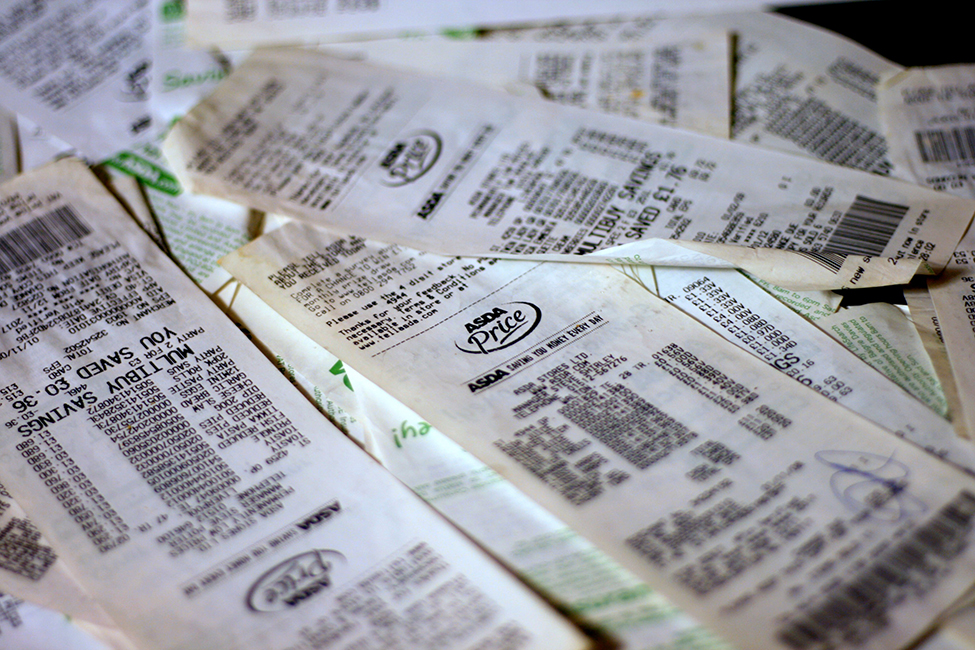The Chi-Square Distribution

# 55 Introduction

The chi-square distribution can be used to find relationships between two things, like grocery prices at different stores. (credit: Pete/flickr)Have you ever wondered if lottery winning numbers were evenly distributed or if some numbers occurred with a greater frequency? How about if the types of movies people preferred were different across different age groups? What about if a coffee machine was dispensing approximately the same amount of coffee each time? You could answer these questions by conducting a hypothesis test.

You will now study a new distribution, one that is used to determine the answers to such questions. This distribution is called the chi-square distribution.

In this chapter, you will learn the three major applications of the chi-square distribution:

1. the goodness-of-fit test, which determines if data fit a particular distribution, such as in the lottery example
2. the test of independence, which determines if events are independent, such as in the movie example
3. the test of a single variance, which tests variability, such as in the coffee example